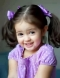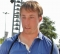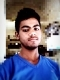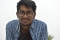## Python program to check whether two lists are circularly identicalPublished on 26-Sep-2018 16:08:59
Here two lists are given. Our task is to check and found weather two given lists are circularly identical or not. Example Input : A = [100, 100, 10, 10, 100] B = [100, 100, 100, 10, 10] Output : True ... Read More

## C++ Program To Convert Decimal Number to BinaryPublished on 26-Sep-2018 16:08:59
In a computer system, the binary number is expressed in the binary numeral system while the decimal number is in the decimal numeral system. The binary number is in base 2 while the decimal number is in base 10. Examples of decimal numbers and their corresponding binary numbers are as ... Read More

## C++ Program to Print Number Entered by UserPublished on 26-Sep-2018 16:03:35
The objects “cin” and “cout” are used in C++ for input and output respectively. cin is an instance of the istream class and is attached to the standard input device such as the keyboard. cout is an instance of the ostream class and is connected to the standard output device ... Read More

## C++ Program to Check Whether a Number is Prime or NotPublished on 26-Sep-2018 16:00:08
A prime number is a whole number that is greater than one and the only factors of a prime number should be one and itself. Some of the first prime numbers are −2, 3, 5, 7, 11, 13 ,17The program to check if a number is prime or not is ... Read More

## Python program to convert a list of tuples into DictionaryPublished on 26-Sep-2018 15:53:08
Here one tuple is given, our task is to convert tuples to dictionary. To solve this problem we use the dictionary method setdefault (). This method has two parameter, to convert the first parameter to key and the second to the value of the dictionary. Setdefault (key, value) is a ... Read More

## C++ Program to Display Factors of a NumberPublished on 26-Sep-2018 15:49:45
Factors are those numbers that are multiplied to get a number.For example: 5 and 3 are factors of 15 as 5*3=15. Similarly other factors of 15 are 1 and 15 as 15*1=15.The program to display the factors of a number are given as follows.Example Live Demo#include<iostream> using namespace std; int main() ... Read More

## Python Program to print unique values from a listPublished on 26-Sep-2018 15:43:54
Given a list, our task is to print all the unique numbers.ExampleInput:A = [1, 2, 3, 4, 2, 1, 9] Unique list is [1, 2, 3, 4, 9]AlgorithmStep 1: Create user input list. Step 2: Create an empty list. Step 3: Traverse all elements in the list. Step 4: Check ... Read More

## C++ Program to Display Fibonacci SeriesPublished on 26-Sep-2018 15:42:25
The fibonacci series contains numbers in which each term is the sum of the previous two terms. This creates the following integer sequence −0, 1, 1, 2, 3, 5, 8, 13, 21, 34, 55, 89, 144, 233, 377…….The recurrence relation that defines the fibonacci numbers is as follows −F(n) = ... Read More

## Python program to check if all the values in a list that are greater than a given valuePublished on 26-Sep-2018 15:33:25
List is given and checking value is given, display all the values in a list that are greater than the given value.ExampleInput : A=[10, 20, 30, 40, 50] Given value=20 Output : No Input : A=[10, 20, 30, 40, 50] Given value=5 Output : YesAlgorithmStep 1: Create user input list. ... Read More

## C++ "Hello, World!" ProgramPublished on 26-Sep-2018 15:33:28
C++ is a general purpose programming language that supports procedural, object-oriented and generic programming. C++ is a superset of C and all valid C programs are valid in C++ as well.C++ supports object oriented programming with features such as data hiding, encapsulation, inheritance, polymorphism etc.Let us see the first C++ ... Read More